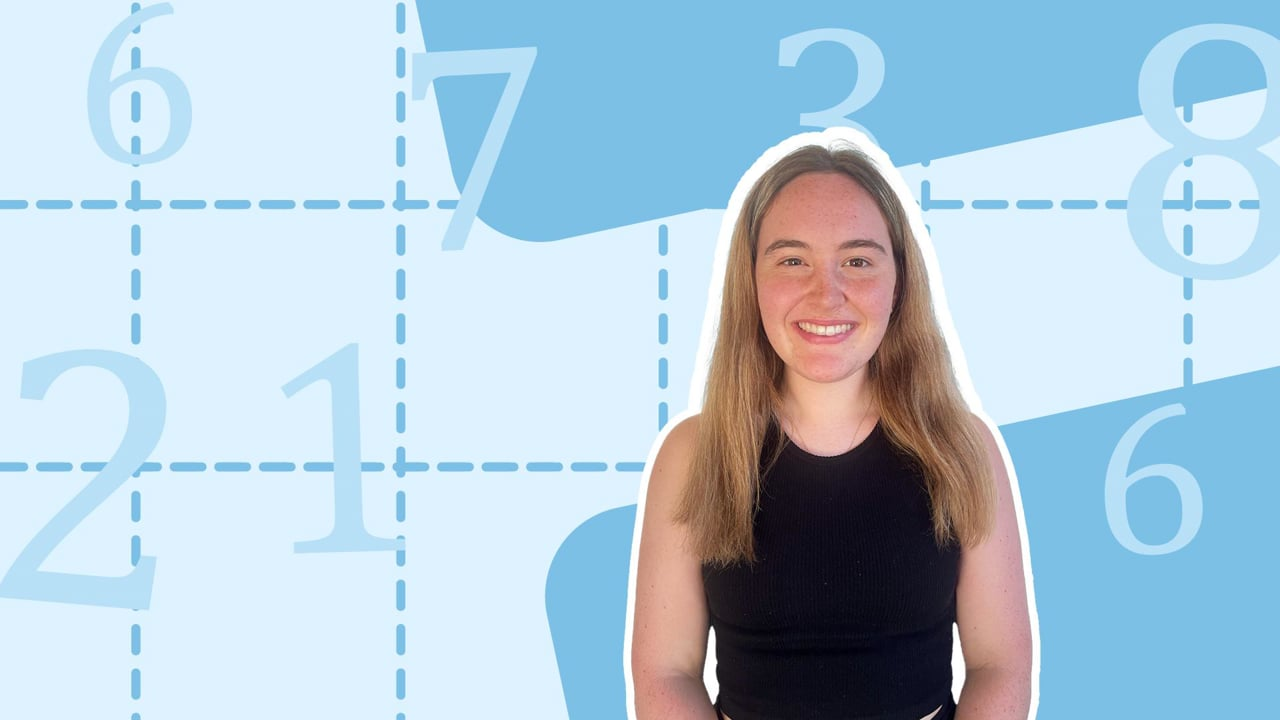Chapter overviewMaths

Number and place value

Multiplication and division

Fractions

Measurement

Geometry - properties of shapes

Statistics

Maths

# Counting in multiples of 4, 8, 50 and 1000%

Summary

# Counting in multiples of 4, 8, 50 and 100

## ​​In a nutshell

Counting in multiples of $4,8,50$ and $100$ is similar to recalling times tables. There are patterns that can be recognised to make multiples easier remember and use.

## What is a multiple?

### ​​Definition

A multiple is the result of multiplying two numbers together. Counting in multiples means starting from zero and adding the same number each time. It is the easiest way to see multiples and the patterns that they produce.

### Multiples of 4

To find the multiples of $4$, create a sequence and add four to each number, starting from zero.

$0 \xrightarrow[]{+4} \underline4 \xrightarrow[]{+4} \underline8\xrightarrow[]{+4} \underline{12}\xrightarrow[]{+4}\underline{16}$

All the multiples of $4$ up to $48$ are listed below.

$4,8,12,16,20,24,28,32,36,40,44,48$

Patterns to notice about multiples of $4$​:

• They are all even: each multiple of $4$ ends in $2,4,6,8$ or $0$.
• They are all multiples of two and can be halved twice.

### Multiples of 8

Multiples of $8$ are an extension of the eight times tables. To find the multiples of $8$, create a sequence and add eight to each number, starting from zero.

$0 \xrightarrow[]{+8} \underline8 \xrightarrow[]{+8} \underline{16}\xrightarrow[]{+8} \underline{24}\xrightarrow[]{+8}\underline{32}$

All the multiples of $8$​ up to $96$ are listed below.

$8,16,24,32,40,48,56,64,72,80,88,96$

Patterns to notice about multiples of $8$​:

• They are all even: each multiple of eight ends in $2,4,6,8$ or $0$.
• They are double the multiples of $4$.

Tip: Notice that the end digits of multiples follow a pattern themselves, for example here they follow the order $8,6,4,2,0$. Use this to check the next number in the sequence!

### Multiples of 100

Counting in multiples of $100$ is very similar to counting in multiples of $10$, except there is an extra zero on the end.

Multiples of $10$:

$0 \xrightarrow[]{+10} \underline{10} \xrightarrow[]{+10} \underline{20}\xrightarrow[]{+10} \underline{30}\xrightarrow[]{+10}\underline{40}$

Multiples of $100$

$0 \xrightarrow[]{+100} \underline{100} \xrightarrow[]{+100} \underline{200}\xrightarrow[]{+100} \underline{300}\xrightarrow[]{+100}\underline{400}$

This is because $100$ is ten lots of $10$.

All the multiples of $100$​ up to $1000$ are listed below.

$100,200,300,400,500,600,700,800,900,1000$

The multiples of $100$ are read as 'one hundred', 'two hundred',...'nine hundred', 'one thousand', 'one thousand, one hundred'...

Patterns to notice about multiples of $100$​:

• They all end in $00$ .
• They are all multiples of ten: each multiple of $100$​ ends in $0$.

### Multiples of 50

Counting in multiples of $50$ is very similar to counting in multiples of $5$, except there is an extra zero on the end.

Multiples of $5$:

$0 \xrightarrow[]{+5} \underline5 \xrightarrow[]{+5} \underline{10}\xrightarrow[]{+5} \underline{15}\xrightarrow[]{+5}\underline{20}$

Multiples of $50$

$0 \xrightarrow[]{+50} \underline{50} \xrightarrow[]{+50} \underline{100}\xrightarrow[]{+50} \underline{150}\xrightarrow[]{+50}\underline{200}$

This is because $50$ is ten lots of $5$.

All the multiples of $50$​ up to $500$ are listed below.

$50,100,150,200,250,300,350,400,450,500$

Patterns to notice about multiples of $50$​:

• They all end in either $00$ or $50$.
• They are all multiples of ten: each multiple of $50$ ends in $0$.

Tip: Another way to count in multiples of $50$ is to add $10$ five times!

FAQs

• Question: How to spot multiples of 50?

Answer: There are a couple of patterns to help you spot multiples of 50​:​ 1. They all end in either 00 or 50. 2. They are all multiples of ten: each multiple of 50 ends in 0.

• Question: How to spot multiples of 4?

Answer: There are a couple of patterns to help you spot a multiple of 4: 1. They are all even: each multiple of 4 ends in 2, 4, 6, 8 or 0. 2. They are all multiples of two and can be or halved twice.

• Question: What is a multiple?

Answer: Multiples are extensions of times tables. Counting in multiples means starting from zero and adding the same number each time. It is the easiest way to see multiples and the patterns that they produce.

Theory

Exercises